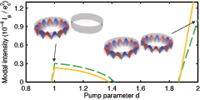September 2011
Mon Tue Wed Thu Fri Sat Sun
« Aug   Oct »
1234
567891011
12131415161718
19202122232425
2627282930

## Hermitian scattering behavior for the non-Hermitian scattering center

L. Jin, Z. Song

We study the scattering problem for the non-Hermitian scattering center, which consists of two Hermitian clusters with anti-Hermitian couplings between them. Counterintuitively, it is shown that it acts as a Hermitian scattering center, satisfying $$|r| ^{2}+|t| ^{2}=1$$, i.e., the Dirac probability current is conserved, when one of two clusters is embedded in the waveguides. This conclusion can be applied to an arbitrary parity-symmetric real Hermitian graph with additional PT-symmetric potentials, which is more feasible in experiment. Exactly solvable model is presented to illustrate the theory. Bethe ansatz solution indicates that the transmission spectrum of such a cluster displays peculiar feature arising from the non-Hermiticity of the scattering center.

http://arxiv.org/abs/1109.2187
Quantum Physics (quant-ph)

## A note on topological insulator phase in non-hermitian quantum systems

Pijush K. Ghosh

Examples of non-hermitian quantum systems admitting topological insulator phase are presented in one, two and three space dimensions. All of these non-hermitian Hamiltonians have entirely real bulk eigenvalues and unitarity is maintained with the introduction of appropriate inner-products in the corresponding Hilbert spaces. The topological invariant characterizing a particular phase is shown to be identical for a non-hermitian Hamiltonian and its hermitian counterpart, to which it is related through a non-unitary similarity transformation. A classification scheme for topological insulator phases in pseudo-hermitian quantum systems is suggested.

http://arxiv.org/abs/1109.1697
Quantum Physics (quant-ph); High Energy Physics – Theory (hep-th)

## On the Eigenvalue Density of the non-Hermitian Wilson Dirac Operator

Mario Kieburg, Jacobus J.M. Verbaarschot, Savvas Zafeiropoulos

We find the lattice spacing dependence of the eigenvalue density of the non-Hermitian Wilson Dirac operator in the $$\epsilon$$-domain. The starting point is the joint probability density of the corresponding random matrix theory. In addition to the density of the complex eigenvalues we also obtain the density of the real eigenvalues separately for positive and negative chiralities as well as an explicit analytical expression for the number of additional real modes.

http://arxiv.org/abs/1109.0656
High Energy Physics – Lattice (hep-lat); High Energy Physics – Theory (hep-th); Mathematical Physics (math-ph)

## Non linear pseudo-bosons versus hidden Hermiticity

Fabio Bagarello, Miloslav Znojil

The increasingly popular concept of a hidden Hermiticity of operators (i.e., of their Hermiticity with respect to an {\it ad hoc} inner product in Hilbert space) is compared with the recently introduced notion of {\em non-linear pseudo-bosons}. The formal equivalence between these two notions is deduced under very general assumptions. Examples of their applicability in quantum mechanics are discussed.

http://arxiv.org/abs/1109.0605
Mathematical Physics (math-ph); Functional Analysis (math.FA); Quantum Physics (quant-ph)

## Pump-induced Exceptional Points in Lasers above ThresholdM. Liertzer, Li Ge, A. Cerjan, A. D. Stone, H. E. Türeci, S. Rotter

We demonstrate that the above-threshold behavior of a laser can be strongly affected by exceptional points which are induced by pumping the laser non-uniformly. At these singularities the eigenstates of the non-Hermitian operator which describes the lasing modes coalesce. In the vicinity of these points the laser may turn off even when the overall pump power deposited in the system is increased. We suggest that such signatures of a pump-induced exceptional point can be experimentally probed with coupled ridge or microdisk lasers.

http://arxiv.org/abs/1109.0454
Optics (physics.optics)

http://arxiv.org/abs/1109.0454

## PT Symmetric, Hermitian and P-Self-Adjoint Operators Related to Potentials in PT Quantum Mechanics

Tomas Azizov, Carsten Trunk

In the recent years a generalization $$H=p^2 +x^2(ix)^\epsilon$$ of the harmonic oscillator using a complex deformation was investigated, where $$\epsilon$$ is a real parameter. Here, we will consider the most simple case: $$\epsilon$$ even and $$x$$ real. We will give a complete characterization of three different classes of operators associated with the differential expression H: The class of all self-adjoint (Hermitian) operators, the class of all PT symmetric operators and the class of all P-self-adjoint operators. Surprisingly, some of the PT symmetric operators associated to this expression have no resolvent set.

http://arxiv.org/abs/1108.5923
Quantum Physics (quant-ph)

## Origin of maximal symmetry breaking in even PT-symmetric lattices

Yogesh N. Joglekar, Jacob L. Barnett

By investigating a parity and time-reversal (PT) symmetric, $N$-site lattice with impurities $$\pm i\gamma$$ and hopping amplitudes $$t_0 (t_b)$$ for regions outside (between) the impurity locations, we probe the origin of maximal PT-symmetry breaking that occurs when the impurities are nearest neighbors. Through a simple and exact derivation, we prove that the critical impurity strength is equal to the hopping amplitude between the impurities, $$\gamma_c=t_b$$, and the simultaneous emergence of $$N$$ complex eigenvalues is a robust feature of any PT-symmetric hopping profile. Our results show that the threshold strength $$\gamma_c$$ can be widely tuned by a small change in the global profile of the lattice, and thus have experimental implications.

http://arxiv.org/abs/1108.6083
Quantum Physics (quant-ph); Statistical Mechanics (cond-mat.stat-mech)